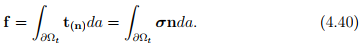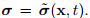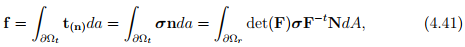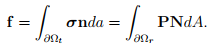# Other Stress Measures Civil Engineering (CE) Notes | EduRev

## Civil Engineering (CE) : Other Stress Measures Civil Engineering (CE) Notes | EduRev

The document Other Stress Measures Civil Engineering (CE) Notes | EduRev is a part of the Civil Engineering (CE) Course Advanced Solid Mechanics - Notes, Videos, MCQs & PPTs.
All you need of Civil Engineering (CE) at this link: Civil Engineering (CE)

Other stress measures
Till now, we have been looking only at the Cauchy (or true) stress. As its name suggest this is the “true” measure of stress. However, there are many other definition of stresses but all of these are propounded just to facilitate easy algebra. Except for the first Piola-Kirchhoff stress, other stress measures do not have a physical interpretation as well.

Piola-Kirchhoff stress tensors
Let ∂Ωt denote some surface within or on the boundary of the body in the current configuration. Then, the net force acting on this surface is given byIt is easy to compute the above integral when the Cauchy stress is expressed as a function of x, the position vector of a typical material point in the current configuration, i.e.On the other hand computing the above integral becomes difficult when the stress is a function of X, the position vector of a typical material point in the reference configuration, i.e.To facilitate the computation of the above integral in the later case, we appeal to the Nanson’s formula (3.72) to obtainwhere ∂Ωr is the surface in the reference configuration, formed by the same material particles that formed the surface ∂Ωt in the current configuration. Then, the first Piola-Kirchhoff stress, P is defined as

P = det(F)σF−t .                        (4.42)

Using this definition of Piola-Kirchhoff stress, equation (4.41) can be written as(4.43)

Assuming, the stress to be uniform over the surface of interest and the state of stress corresponds to pure normal stress, it is easy to see that while Cauchy stress is force per unit area in the current configuration, Piola-Kirchhoff stress is force per unit area in the reference configuration. This is the essential difference between the Piola-Kirchhoff stress and Cauchy stress. Recognize that in both the cases the force acts only in the current configuration. In lieu of this Piola-Kirchhoff stress is a two-point tensor, similar to the deformation gradient, in that it is a linear transformation that relates the unit normal to the surface in the reference configuration to the traction acting in the current configuration. Thus, if {Ea} denote the three Cartesian basis vectors used to describe the reference configuration and {ea} denote the Cartesian basis vectors used to describe the current configuration, then the Cauchy stress and Piola-Kirchhoff stress are represented as

σ = σabea ⊗ eb,                              P = PaBeaEB.                      (4.44)

We had mentioned earlier that the Cauchy stress tensor is symmetric for reasons to be discussed in the next chapter. Since, σ = PF/ det(F), the symmetric restriction on Cauchy stress tensor requires the Piola-Kirchhoff stress to satisfy the relation

PFt = FPt                              (4.45)

Consequently, in general P is not symmetric and has nine independent components.
During an experiment, it is easy to find the surface areas in the reference configuration and determine the traction or forces acting on these surfaces as the experiment progresses. Thus, Piola-Kirchhoff stress is what could be directly determined. Also, it is the stress that is reported, in many cases. The Cauchy stress is then obtained using the equation (4.42) from the estimate of the Piola-Kirchhoff stress and the deformation of the respective surface. Further, since in solid mechanics, the coordinates of the material particles in the reference configuration is used as independent variable, first PiolaKirchhoff stress plays an important role in formulating the boundary value problem as we shall see in the ensuing chapters.

The transpose of the Piola-Kirchhoff stress, Pt , is called as engineering stress or nominal stress. Another stress measure called the second Piola-Kirchhoff stress, S defined as

S = det(F)F−1σF−t = F−1P                                                                    (4.46)

is used in some studies. Note that this tensor is symmetric and hence its utility.

Kirchhoff, Biot and Mandel stress measures
Next, we define a few stress measures popular in literature. It is easy to establish their inter-relationship and properties which we leave it as an exercise.
The Kirchhoff stress tensor, τ is defined as: τ = det(F)σ.
The Biot stress tensor, TB also called material stress tensor is defined as: TB = RtP, where R is the orthogonal tensor obtained during polar decomposition of F.
The co-rotated Cauchy stress tensor σu, introduced by Green and Naghdi is defined as: σu = RtσR. The Mandel stress tensor, used often to describe inelastic response of materials is defined as Σ = CS, where C is the right Cauchy-Green stretch tensor introduced in the previous chapter.

Offer running on EduRev: Apply code STAYHOME200 to get INR 200 off on our premium plan EduRev Infinity!

## Advanced Solid Mechanics - Notes, Videos, MCQs & PPTs

42 videos|61 docs

,

,

,

,

,

,

,

,

,

,

,

,

,

,

,

,

,

,

,

,

,

;